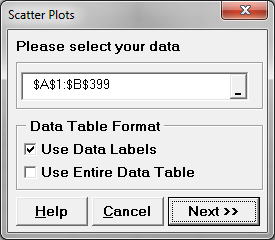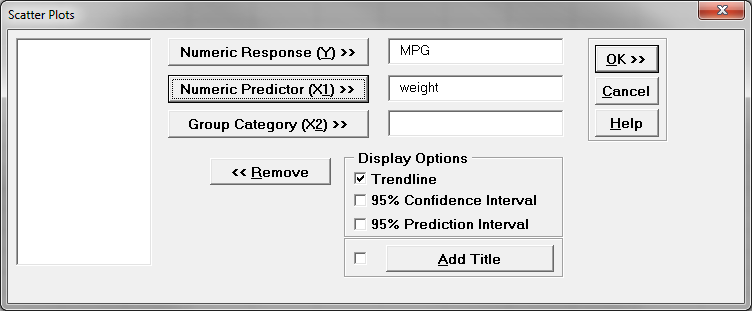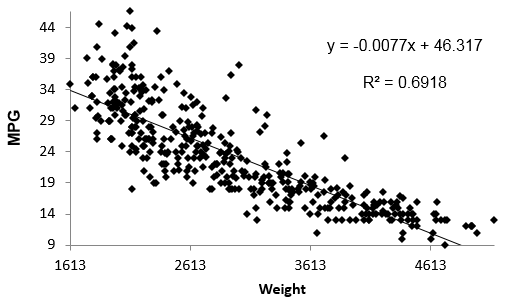# Scatter Plot with SigmaXL

### What is a Scatter Plot

A scatter plot is a diagram to present the relationship between two variables of a data set. It consists of a set of data points set on two axis. On the scatter plot, a single observation is presented by a data point with its horizontal position equal to the value of one variable and its vertical position equal to the value of the other variable. A scatter plot helps us to understand:

• Whether the two variables are related to each other or not
• What is the strength of their relationship
• The shape of their relationship
• The direction of their relationship
• Whether outliers are present

### How to Use SigmaXL to Generate a Scatter Plot

Data File: “Scatter Plot” tab in “Sample Data.xlsx”

Steps to render a Scatterplot in SigmaXL:

1. Select the entire range of data (both “MPG” and “weight”)
2. Click SigmaXL -> Graphical Tools -> Scatter Plots
3. A new window named “Scatter Plots” pops up with the selected range of data appearing in the box under “Please select your data”4. Click “Next>>”
5. A new window also named “Scatter Plots” appears
6. Select “MPG” as the “Numeric Response (Y)”
7. Select “weight” as the “Numeric Predictor (X1)”8. Click “OK>>”
9. The scatterplot appears in the new tab “Scatterplot (1)”Model summary: The figure above is SigmaXL’s output of the scatterplot data. You can immediately see the value of graphical displays of data. This information obtainable by viewing this output shows a relationship between weight and MPG. This scatterplot shows that the heavier the weight the lower the MPG value and vice versa.